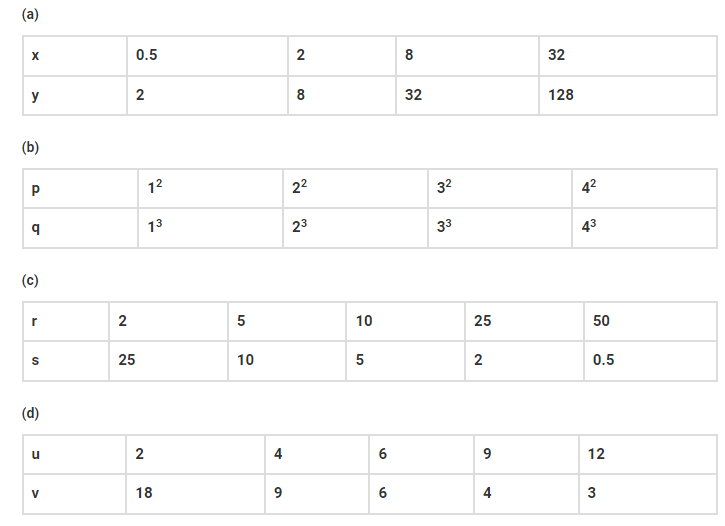# In which of the following case,`
Question:

In which of the following case, do the quantities vary directly with each other?Solution:

Option (a)

Explanation: In option (a), the values of x is directly proportional to values of y, such as;

y = 4x

If we put the values of x = 0.5, 2, 8 and 32, we get the values of y as 2, 8, 32 and 128 respectively.